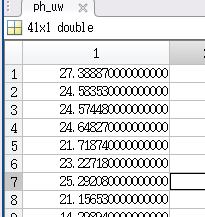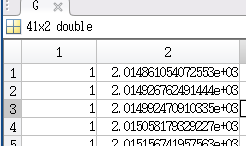# StaMPS-Visualizer, SNAP-StaMPS Workflow

what’s wrong with his answer here? Documentation on SNAP-StaMPS workflow

1 Like

Nothing wrong Dear ABraun, In this below link vertical_displacement_calculator.py code is present. I want to check it this python wrapper to descending orbit data set.

Thank you.

gjvanleeuwen/SNAP-StaMPS-Visualizing: Documentation on the bachelor thesis: AN ASSESMENT OF SENTINEL-1 RADAR IMAGERY TIME-SERIES ANAYLIS FOR MONITORING SEASONAL SURFACE SUBSIDENCE DUE TO PERMAFROST DEGRADATION AFTER A TUNDRA FIRE (github.com)

I have used the csv file obtained by StaMPS-Vsualizer. How does StaMPS calculate the average deformation rate based on the settlement?

To which column do you refer to?

The third column，mean velocity

As far as I understand it, over the entire time period of your stack (lets say its 2 years for example), the velocity is calculated by looking at the absolute difference between the first and last point (lets say for one point that is 4mm) then you have a 2mm/yr velocity.

However, there might be other ways to calculate that. e.g. calculating the velocity for the period 1st image to prime and a second velocity for prime to last image and then the mean of both results. But as far as I remember (I looked into this in 2017/2018) It was done as described in the first option

Yes sir, you made a correct point, but there are still some differences between the average speed calculated by the first method and the value directly derived from StaMPS. I don’t know where these differences come from. Do you have any good suggestions for this, thank you！

No other than the two options I mentioned. But I looked into the MATLAB code of StaMPS and localized the lines where mean velocity is calculated:

some objects are prepraed before but the main part is from line 196 to 204:

As you can see, it is not that straight forward as described, which explaines the little differences. If you like read the script closely and when you post a summary here about how exactly the mean velocity is calculated we would all be very thankful2 Likes

Sir, I did some tests with the exported data according to the code in ps_mean_v.m, and found that the results obtained are satisfactory！

The data in ph_uw represents the cumulative deformation of each imaging time,As shownG represents the matrix of imaging time：the most important is：mean_v=lscov(G,ph_uw,ifg_cov)’;Since I don’t know what ifg_cov represents, So I use mean_v=lscov(G,ph_uw)’; command to test.

The results show that the difference between the calculated average deformation rate and the result derived by StaMPS is less than 1

Finally, attach a description of the lscov function in matlab:

3 Likes

@weiweian Thanks for sharing your insights! That will help others to understand this value better. The question about the precise calculation of mean velocity came already up once or twice.

1 Like

Dear thho, do you have any idea how I can force 0 to be the mid-point of the colour ramp?

you have to set an area as reference with either

• ref_centre_lonlat: [0 0]

the area you select here will be the zero-displacement reference, so depending on which point you select, this will affect the legend. If nothing is defined, StaMPS takes the average displacement of the scene, but this can differ from the true zero-displacement depending on the size of the scene and the displacement pattern.

if you want to do this in StaMPS-Visualizer, this is tricky I think, here is how it works in general:

• the colorramp is defined by the min and max of your displacement values which you have exported from StaMPS in Matlab.
• Since the distribution can have many characteristics like all positive or negative, 90% over 0 and 10% under 0 (e.g.) the color ramp it is not designed to have a static center but to adjust to every new dataset and give a high resolution of the displacement values aka make a min-max stretch very time
• the line where this is specified is:

here we take the pre defined matlab like color ramp object `colramp` and force the domain to be the min max of the displacement value (which can be seen as a min max stretch)

The new object `colnum` is later used here to set the `pal ` parameter in the map legend:

however, the points color is defined directly via the displacement values:

So what you have to do, in order to center around 0 individually

• create a custom color ramp by stacking two color ramps
• first ramp is e.g. from +10 to 0; blue to white
• second ramp is e.g. from 0 to -90; white to red
• stack both color ramps to gain a +10 - 0 - -90; blue - white - red gradient color ramp
• then, this new color ramp has to be implemented to the two cases (legend color and point color)…how to do this exactly, I have to test myself…tbh

here is a code example that can be used, it is somewhat tricky I think, at the moment I do not have the time to implement it, but I will keep it in mind for the future

2 Likes

Hi @ABraun,

if I set ref_centre_lonlat and ref_radius so take a PS as zero-displacement reference (a stable rocky outcrop, for example), when I visualize the graph of this point on StaMPS-Visualizer it will be displayed as a horizontal line at zero (translation of every point data to zero) or only the mean velocity of this PS will be set to zero, or it will affect only the legend but not the data of displacement? Will the velocities of all others PS be affected by the setting of reference point?

Thanks.

yes, the entire range of values will be shifted so that the area you selected as reference is zero. If this value also represents most of the unchanged areas, the legend will also be zero for this color.

2 LikesHi dear thho; when I want to export custom data from stamps based on suggested commands in Github( stamps visualization example); I get this attached error…what does it mean?

Hi @zahra0729,

the script is missing an object called ref_centre_lonlat.

If I remember right, this object is created during the line `load parms.mat` in the beginning of the script. You normally set it at the beginning of StamPS processing to define a region that is assumed to be stable and which phase values are used for reference (otherwise all displacement values are relative to a mean made from all values…which might cause strange results). However, normally, if the parameter was not set in StaMPS it should be 0,0 or something.

To solve the issue, start the script and run it line by line. In Matlab, track the objects which are created in your environment and check if the object is created properly, and also check if after each line all results are as expected…the custom export script is not the most stable lines of code I have ever written…so I advice to run it line by line and check its behaviour on your specific data.

thanks for your kind response, I could solve it, but when I try to visualize some case study results by this app, there is not a base map in the background of my ps points figure window. what can be the reason for this issue?

First, you should switch to the latest release which I moved to github, it is more stable than the very early version you are using right now. Also installation is very easy with the new version.

The reason for your white background can be caused by a too high zoom level. The newer version of the app has a layer option in the upper left corner of the map panel, there you can switch to OSM basemap which sometimes provide a higher zoom level. If you dig into the code, you can add other WMS tiles as basemap here:

thanks a bunch. I will try it